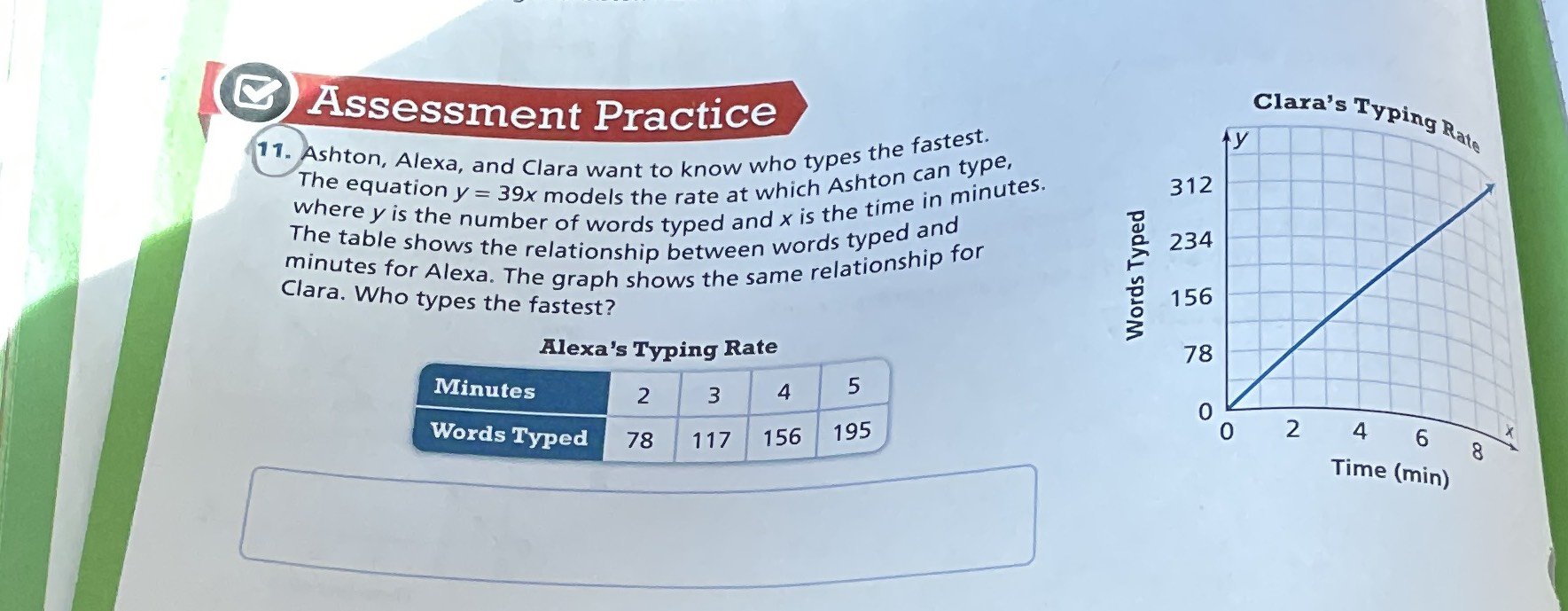### ¿Todavía tienes preguntas de matemáticas?

Pregunte a nuestros tutores expertos
Algebra
Pregunta11. Ashton, Alexa, and Clara want to know who types the fastest. The equation $$y = 39 x$$ models the rate at which Ashton can type, where $$y$$ is the number of words typed and $$x$$ is the time in minutes. The table shows the relationship between words typed and minutes for Alexa. The graph shows the same relationship for Clara. Who types the fastest?